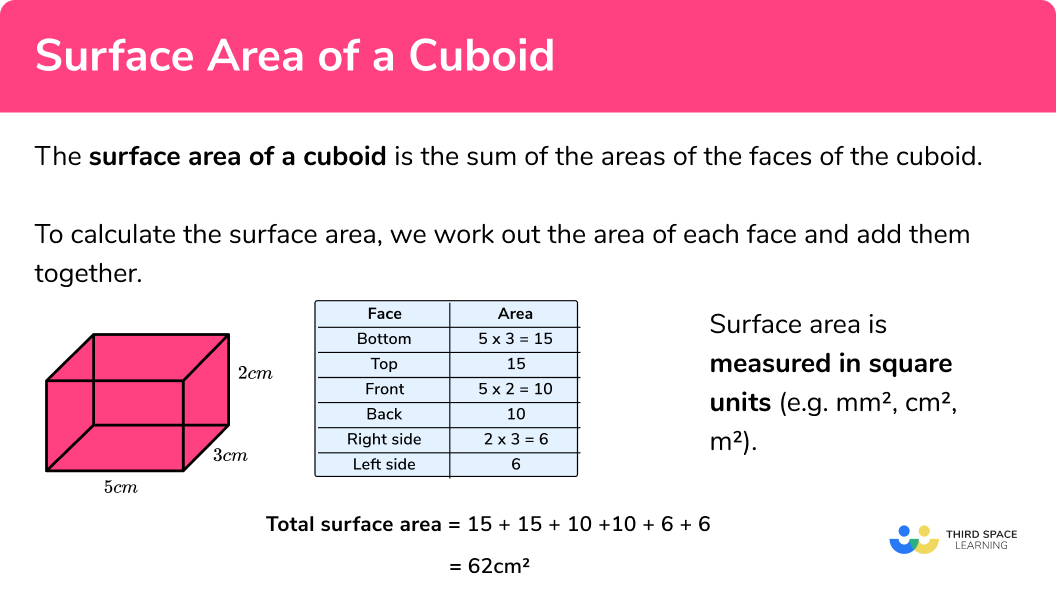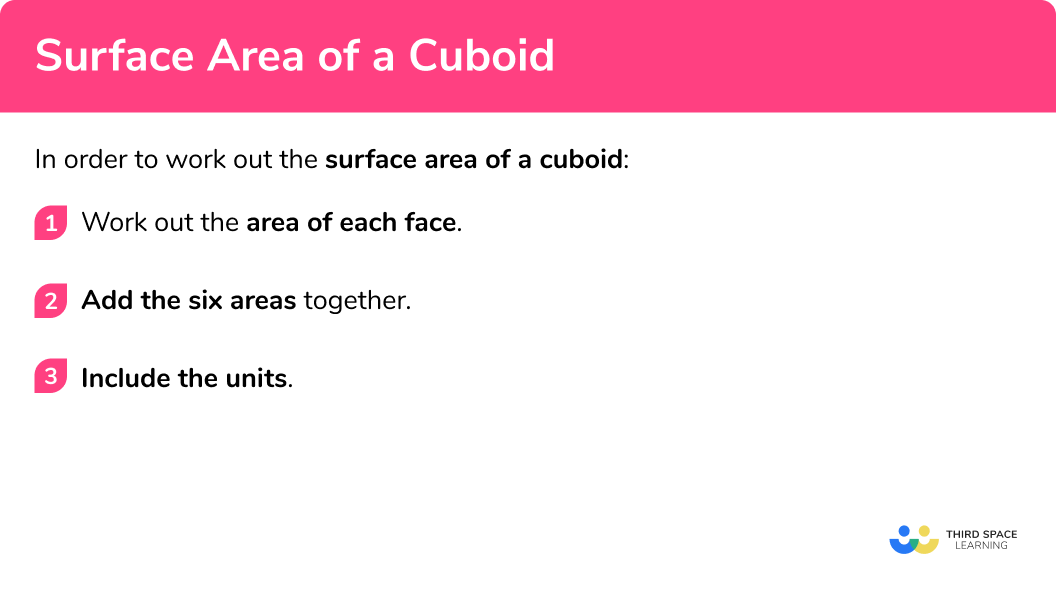GCSE Maths Geometry and Measure 3D Shapes Cuboid

Surface Area Of A Cuboid

# Surface Area Of A Cuboid

Here we will learn about the surface area of a cuboid and how to calculate it.

There are also volume and surface area of a cuboid worksheets based on Edexcel, AQA and OCR exam questions, along with further guidance on where to go next if you’re still stuck.

## What is the surface area of a cuboid?

The surface area of a cuboid is the total area of all of the faces of a cuboid (or rectangular prism).

The 3 dimensions of a cuboid are width, length and height.

Cuboids have three pairs of identical faces – top and bottom, front and back, and left and right.

To work out the total surface area of a cuboid, we need to work out the area of each rectangular face and add them all together.

E.g. Find the surface area of a cuboid.

Since it is an area, surface area is measured in square units (e.g. mm^2, cm^2, m^2 etc).

### What is the surface area of a cuboid?## How to calculate the surface area of a cuboid

In order to work out the surface area of a cuboid:

1. Work out the area of each face.
2. Add the six areas together.
3. Include the units.

### How to calculate the surface area of a cuboid## Surface area of a cuboid examples

### Example 1: surface area of a cuboid

Work out the surface area of the cuboid

1. Work out the area of each face.

The area of the bottom is 9\times3=27cm^2 .

The top face is the same as the bottom face so the area of the top is also 27cm^2 .

The area of the front is 9\times4=36cm^2 .

The back face is the same as the front face so the area of the back is also 36cm^2 .

The area of the right hand side is 3\times4=12cm^2 .

The left side face is the same as the right side face so the area of the left side is also 12cm^2 .

It will make our working clearer if we use a table:

The sum of the areas is: 27+27+36+36+12+12=150

3Include the units.

The measurements on the cuboid are in cm therefore the total surface area of the cuboid = 150cm^2 .

### Example 2: surface area of a cuboid

Work out the surface area of the cuboid

Work out the area of each face.

Include the units.

### Example 3: surface area of a cube

Work out the surface area of this cube

Work out the area of each face.

Include the units.

### Example 4: surface area with different units

Work out the surface area of this cuboid

Work out the area of each face.

Include the units.

### Example 5: surface area using algebra

Work out the surface area of this cuboid.

Work out the area of each face.

Include the units.

### Example 6: surface area using algebra

Work out the surface area of this cuboid

Work out the area of each face.

Include the units.

### Common misconceptions

• Calculating volume instead of surface area

Volume and surface area are different things – volume is the space within the shape whereas surface area is the total area of the faces. To find surface area, we need to work out the area of each face and add them together.

• Equal faces

A common mistake is to think that four of the faces are equal.

E.g.
The first pair of faces are equal to each other.
The second pair of faces are equal to each other.
The third pair of faces are equal to each other.

Surface area of a cuboid is part of our series of lessons to support revision on cuboid. You may find it helpful to start with the main cuboid lesson for a summary of what to expect, or use the step by step guides below for further detail on individual topics. Other lessons in this series include:

### Practice surface area of a cuboid questions

1. Work out the surface area of the cuboid315\mathrm{cm}^{2}222\mathrm{cm}^{2}342\mathrm{cm}^{2}462\mathrm{cm}^{2}Work out the area of each of the six faces:

 Face Area Bottom 7×15=105 Top 105 Front 3×15=45 Back 45 Right side 3×7=21 Left side 21

\text{Total surface area: }105+105+45+45+21+21=342\mathrm{cm}^{2}

2. Work out the surface area of this cube96\mathrm{cm}^{2}64\mathrm{cm}^{2}24\mathrm{cm}^{2}256\mathrm{cm}^{2}Since it is a cube, all of the faces are the same. The area of each face is 4\times 4=16\mathrm{cm}^{2} . There are six identical faces therefore the total surface area of the cube is 6 \times 16=96 \mathrm{cm}^{2}

3. Work out the surface area of this cuboid33.56\mathrm{cm}^{2}4.2\mathrm{cm}^{2}8900\mathrm{cm}^{2}12400\mathrm{cm}^{2}Some of the measurements are in m and one is in cm. We need all of the measurements to be in the same units so convert the metres to centimetres. 0.7m=70cm and 0.4m=40cm . Now we can calculate the areas:

 Face Area Bottom 70×15=1050 Top 1050 Front 70×40=2800 Back 2800 Right side 40×15=600 Left side 600

\text{Total surface area: } 1050+1050+2800+2800+600+600=8900\mathrm{cm}^{2}

4. Work out the surface area of this cuboid16a \mathrm{cm}^{2}15a \mathrm{cm}^{2}(20a+30) \mathrm{cm}^{2}(16a+30) \mathrm{cm}^{2}Work out the area of each of the six faces:

 Face Area Bottom 5×a=5a Top 5a Front 3×a=3a Back 3a Right side 3×5=15 Left side 15

\text{Total surface area: } 5a+5a+3a+3a+15+15=(16a+30) \mathrm{cm}^{2}

5. Work out the surface area of the cuboid80b \mathrm{mm}^{2}16b^{2} \mathrm{mm}^{2}(4b^{2}+48b) \mathrm{mm}^{2}(8b^{2}+16b) \mathrm{mm}^{2}Work out the area of each of the six faces:

 Face Area Bottom 8×b=8b Top 8b Front b×2b=2b2 Back 2b2 Right side 8×2b=16b Left side 16b

\text{Total surface area: }8b+8b+2b^{2}+2b^{2}+16b+16b=(4b^{2}+48b)mm^{2}

6. Given that the surface area of this cuboid is 142\mathrm{cm}^{2} find the value of x .6.76cm5cm14.2cm20.29cmWork out the area of each of the six faces:

 Face Area Bottom 7×x=7x Top 7x Front 3×x=3x Back 3x Right side 3×7=21 Left side 21

\text{Total surface area: }7x+7x+3x+3x+21+21=20x+42

Since we know the surface area is  142\mathrm{cm}^{2}
we can say:
20x+42=142\\ 20x=100\\ x=5\mathrm{cm}

### Surface area of a cuboid GCSE questions

1. Calculate the surface area of the cuboid.(3 marks)

Two of:
12\times 3.5=42\\ 12\times 4=48\\ 4\times 3.5=14

(1)

42+42+48+48+14+14

(1)

208\mathrm{cm}^{2}

(1)

2. A breakfast cereal producer wants to produce a cereal box with a volume of 4800cm^3 . The company wants to use as little cardboard as possible for each box. Should they use box A or box B ? You must show your working.(5 marks)

Box A surface area: 160+160+600+600+240+240

(1)

\text{Surface area }=2000\mathrm{cm}^{2}

(1)

Box B surface area: 192+192+480+480+250+250

(1)

\text{Surface area }=1844\mathrm{cm}^{2}

(1)

They should use box B

(1)

3. John wants to paint 4 identical doors, as shown below.1 litre of paint will cover
10\mathrm{m}^{2}
John has 1 litre of paint. Does he have enough paint to cover all 4 doors? You must show your working.

(5 marks)

5cm = 0.05m

(1)

Surface area of 1 door: 0.03+0.03+1.2+1.2+0.1+0.1

(1)

\text{Surface area of 1 door }=2.66\mathrm{m}^{2}

(1)

\text{Surface area of 4 doors: }4 \times 2.66=10.64\mathrm{m}^{2}

(1)

No he does not have enough paint

(1)

## Learning checklist

You have now learned how to:

• Calculate the surface area of a cuboid
• Use the properties of faces, surfaces, edges and vertices of cubes and cuboids to solve problems in 3D

## Still stuck?

Prepare your KS4 students for maths GCSEs success with Third Space Learning. Weekly online one to one GCSE maths revision lessons delivered by expert maths tutors.

Find out more about our GCSE maths tuition programme.